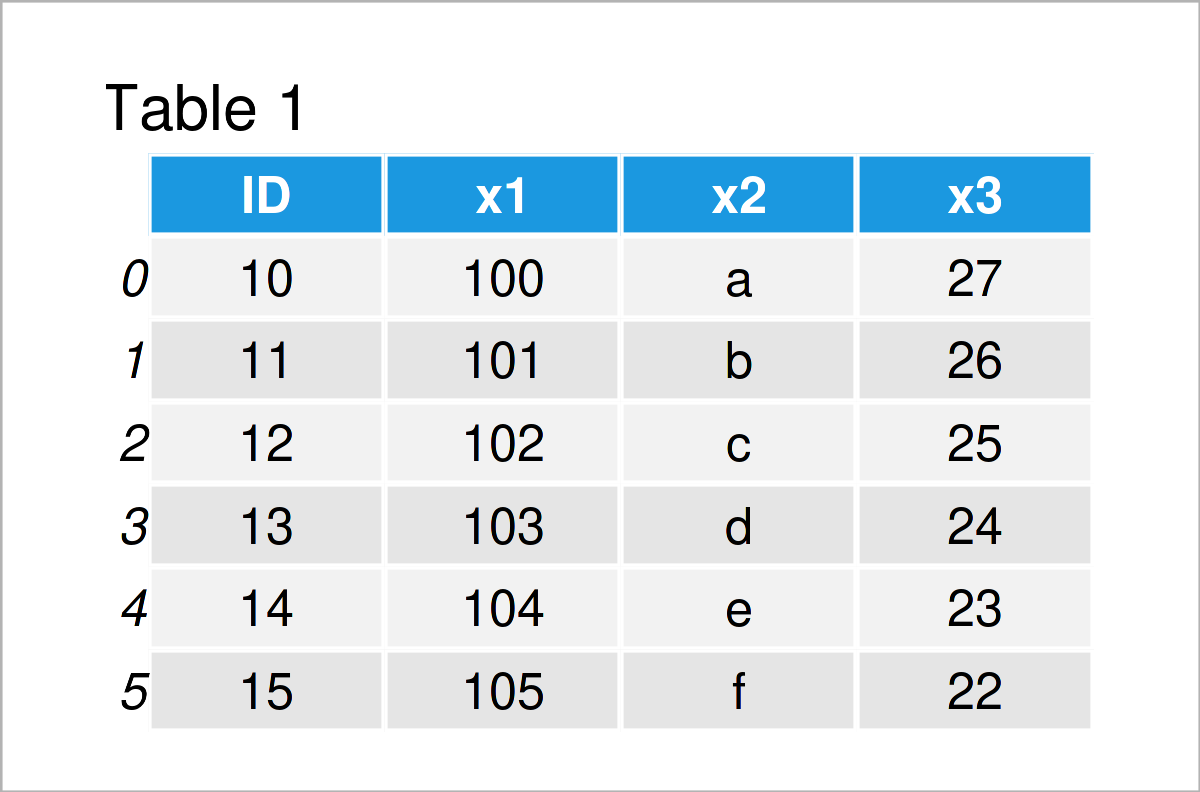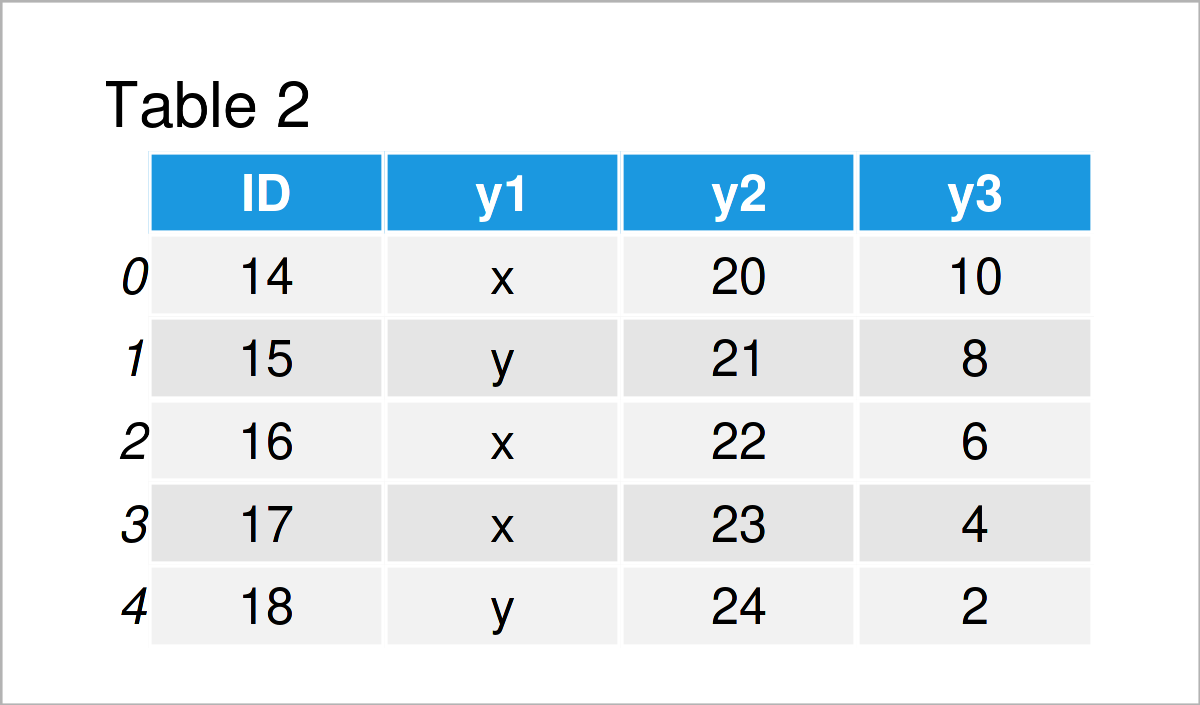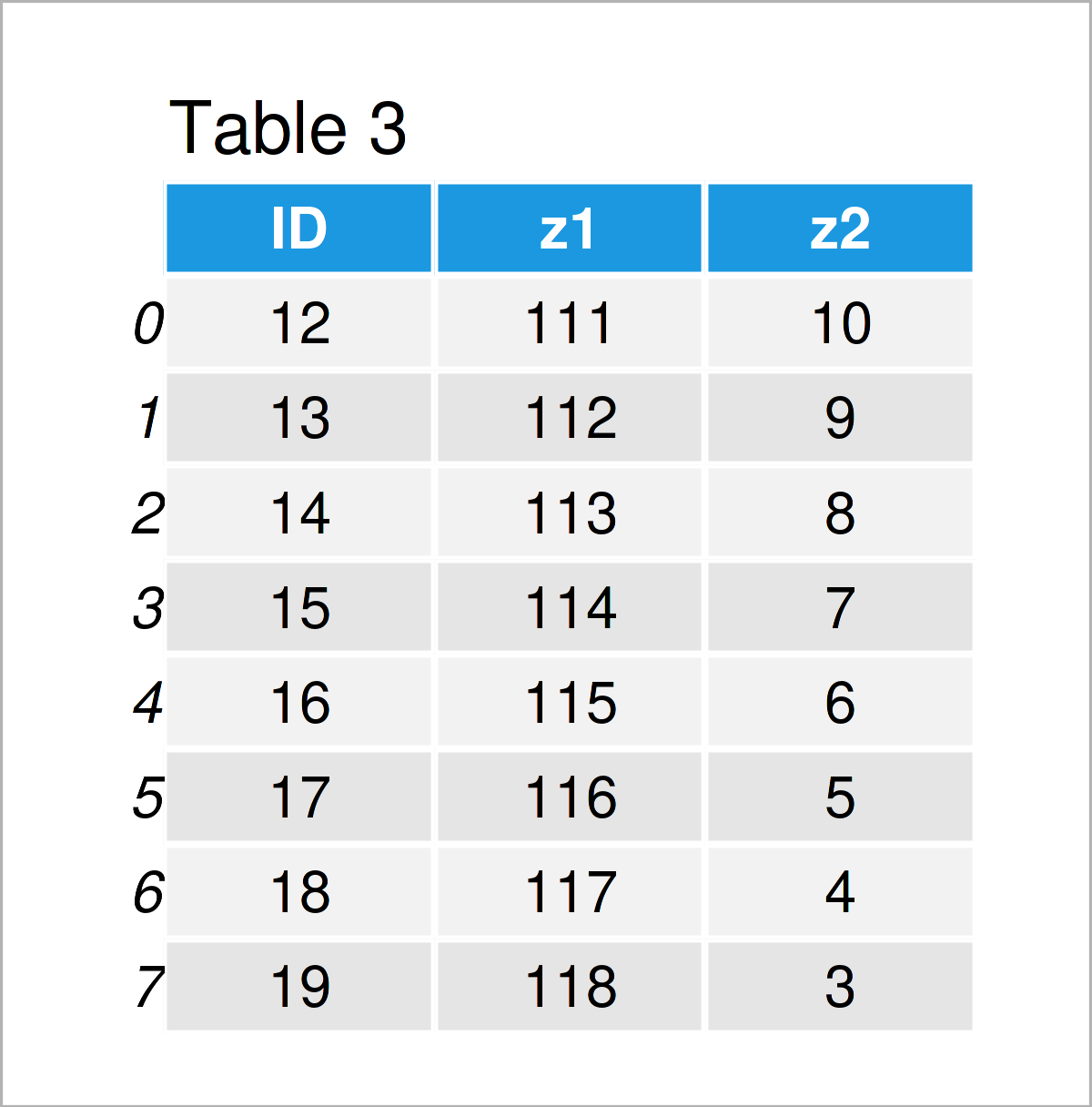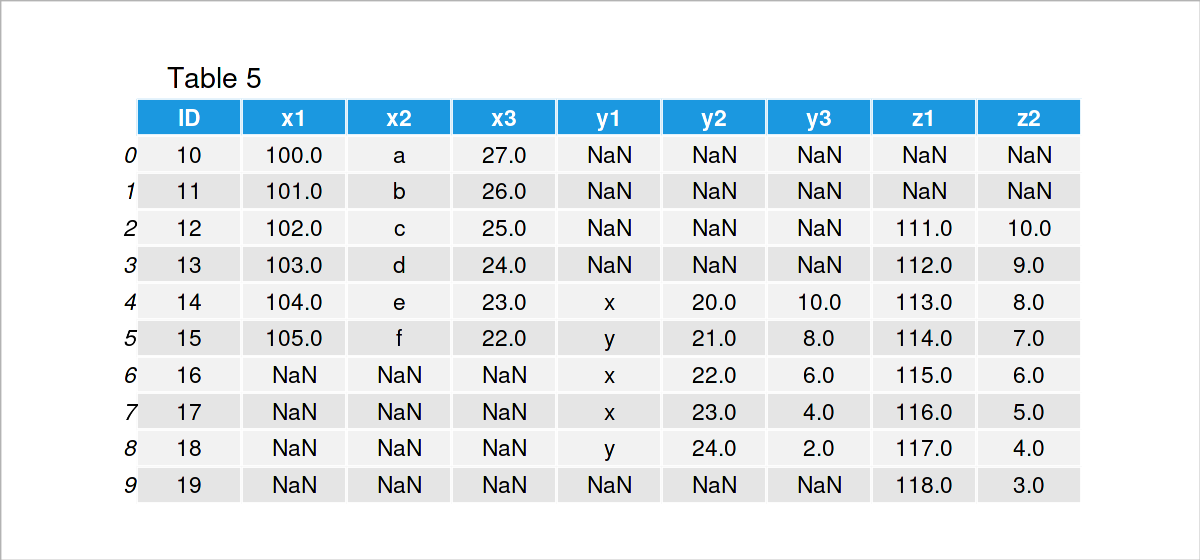# Merge Multiple pandas DataFrames in Python (2 Examples)

In this Python tutorial you’ll learn how to join three or more pandas DataFrames.

Let’s get started:

## Example Data & Software Libraries

We first need to load the pandas library, to be able to use the corresponding functions:

`import pandas as pd                          # Load pandas library`

Let’s also create several example DataFrames in Python:

```data1 = pd.DataFrame({"ID":range(10, 16),    # Create first pandas DataFrame
"x1":range(100, 106),
"x2":["a", "b", "c", "d", "e", "f"],
"x3":range(27, 21, - 1)})
print(data1)                                 # Print first pandas DataFrame``````data2 = pd.DataFrame({"ID":range(14, 19),    # Create second pandas DataFrame
"y1":["x", "y", "x", "x", "y"],
"y2":range(20, 25),
"y3":range(10, 1, - 2)})
print(data2)                                 # Print second pandas DataFrame``````data3 = pd.DataFrame({"ID":range(12, 20),    # Create third pandas DataFrame
"z1":range(111, 119),
"z2":range(10, 2, - 1)})
print(data3)                                 # Print third pandas DataFrame```As shown in Tables 1, 2, and 3, the previous code has created three different pandas DataFrames. All of these DataFrames contain an ID column that we will use to combine the DataFrames in the following examples.

Before we can jump into the merging process, we also have to import the reduce function from the functools module:

`from functools import reduce                 # Import reduce function`

Now, we are set up and can move on to the examples!

## Example 1: Merge Multiple pandas DataFrames Using Inner Join

The following Python programming code illustrates how to perform an inner join to combine three different data sets in Python.

For this, we can apply the Python syntax below:

```data_merge1 = reduce(lambda left, right:     # Merge three pandas DataFrames
pd.merge(left , right,
on = ["ID"]),
[data1, data2, data3])
print(data_merge1)                           # Print merged DataFrame```The output of the previous Python syntax is visualized in Table 4. We have horizontally concatenated our three input DataFrames.

As you can see, we have removed several rows from our data, since we have performed an inner join.

In the next example, I’ll explain how to keep as much data as possible.

## Example 2: Merge Multiple pandas DataFrames Using Outer Join

In Example 2, I’ll show how to combine multiple pandas DataFrames using an outer join (also called full join).

To do this, we have to set the how argument within the merge function to be equal to “outer”:

```data_merge2 = reduce(lambda left, right:     # Merge three pandas DataFrames
pd.merge(left , right,
on = ["ID"],
how = "outer"),
[data1, data2, data3])
print(data_merge2)                           # Print merged DataFrame```After executing the previous Python syntax the horizontally appended pandas DataFrame shown in Table 5 has been created.

This time, we have kept all IDs and rows of our input data sets. For that reason, some of the values in our DataFrame union are NaN.

## Video & Further Resources

Do you need further information on the Python programming code of this tutorial? Then you may want to watch the following video on my YouTube channel. In the video, I’m explaining the content of this article:

Please accept YouTube cookies to play this video. By accepting you will be accessing content from YouTube, a service provided by an external third party.If you accept this notice, your choice will be saved and the page will refresh.

In addition, you might read some of the related tutorials on my website. I have released several tutorials already: